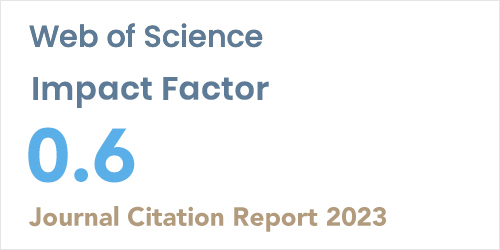ISSN: 1304-7191 | E-ISSN: 1304-7205
Volume: 41 Issue: 4 Year: 2023Data Clustering Based on Fuzzy C-Means and Chaotic Whale Optimization Algorithms
1Computer Engineering Department of Institute of Science, Yildiz Technical University, ISTANBUL
2Computer Engineering Department of Technology Faculty, Duzce University, DUZCE
Sigma J Eng Nat Sci 2019; 37(4): 1107-1128

#### Abstract

Clustering is the process of sub-grouping data according to certain distance and similarity criteria. One of the most commonly used clustering algorithms in the literature is the Fuzzy C-Means (FCM) algorithm based on the fuzzy clustering principle. Although FCM is an efficient algorithm, random selection of initial cluster centers is a disadvantage since it easier trap the algorithm into local optimum. This problem can be solved by approaching the clustering problem as an optimization problem. In this article, Whale Optimization Algorithm (WOA), a global optimization algorithm developed by inspiration from hunting behaviors of humpback whales, has been improved with chaos maps using an adaptive normalization method and chaotic WOA algorithms are proposed. They are then hybridized with FCM algorithm. The performances of the proposed chaotic optimization algorithms are tested with thirteen different benchmark functions. Results are evaluated with means and standard deviations of the objective function values and with the Wilcoxon Sign Rank Test at 0.05 significance level. The clustering performances of the proposed hybrid algorithms measured according to the objective function, the Rand Index and the Adjusted Rand Index values and compared with the K-Means, FCM and some of the other hybrid algorithms for six different data sets selected from the UCI Repository database. In addition, the new hybrid clustering algorithms are improved by using Chebyshev distance function instead of the classical Euclidean distance for the FCM algorithm in order to increase their data clustering performances. As a result, it has been seen that the used chaos functions improve the optimization performance of WOA algorithm, integrating chaotic WOA algorithms with FCM algorithm enhances the disadvantages of FCM algorithm and changing the distance function increases clustering performance of the proposed algorithms.